Let’s continue with the pandas tutorial series. This is the second episode, where I’ll introduce aggregation (such as min, max, sum, count, etc.) and grouping. Both are very commonly used methods in analytics and data science projects – so make sure you go through every detail in this article!

Note 1: this is a hands-on tutorial, so I recommend doing the coding part with me!

## Before we start

If you haven’t done so yet, I recommend going through these articles first:

## Data aggregation – in theory

Aggregation is the process of turning the values of a dataset (or a subset of it) into one single value. Let me make this clear! If you have a DataFrame like…

 animal water_need zebra 100 lion 350 elephant 670 kangaroo 200

…then a simple aggregation method is to calculate the summary of the water_needs, which is 100 + 350 + 670 + 200 = 1320. Or a different aggregation method would be to count the number of the animals, which is 4. So the theory is not too complicated. Let’s see the rest in practice…

## Data aggregation – in practice

Where did we leave off last time? We opened a Jupyter notebook, imported pandas and numpy and loaded two datasets: `zoo.csv` and `article_reads`. We will continue from here – so if you haven’t done the “pandas tutorial – episode 1“, it’s time to go through it!

Okay!
Let’s start with our `zoo` dataset! (If you want to download it again, you can find it at this link.) We have loaded it by using:

`pd.read_csv('zoo.csv', delimiter = ',')`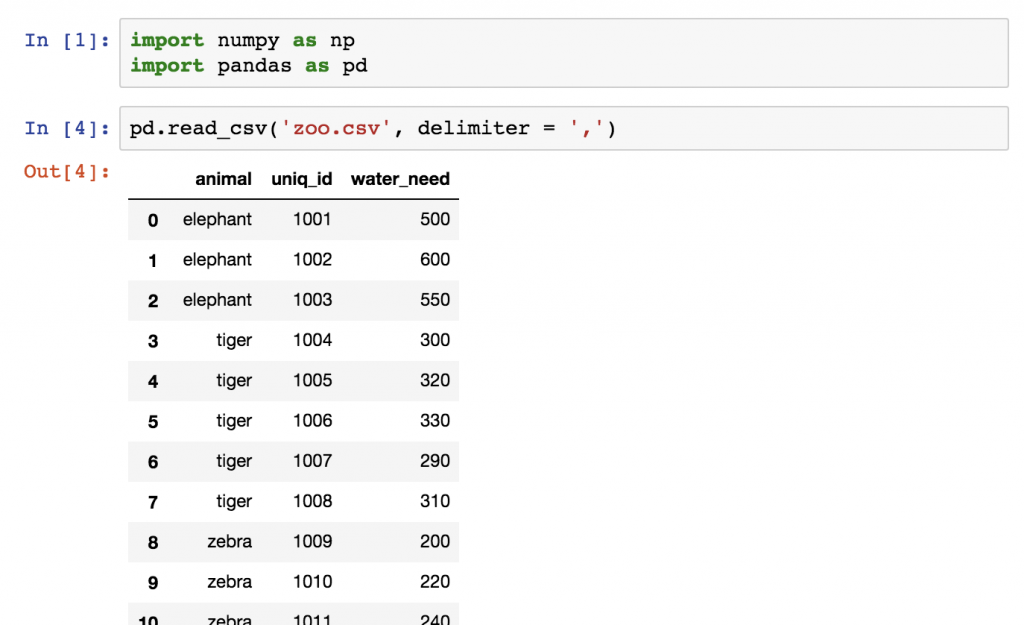Let’s store this dataframe into a variable called `zoo`.

`zoo = pd.read_csv('zoo.csv', delimiter = ',')`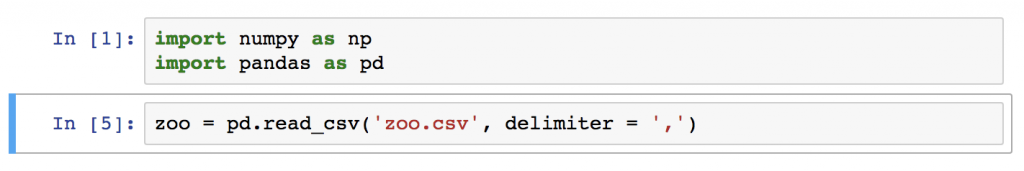Okay, let’s do five things with this data:

1. Let’s count the number of rows (the number of animals) in `zoo`!
2. Let’s calculate the total `water_need` of the animals!
3. Let’s find out which is the smallest `water_need` value!
4. And then the greatest `water_need` value!
5. And eventually the average `water_need`!

## Pandas Data Aggregation #1: .count()

Counting the number of the animals is as easy as applying a count function on the `zoo` dataframe:

`zoo.count()`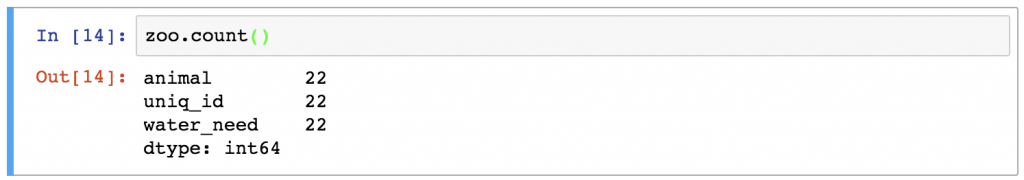Oh, hey, what are all these lines? Actually, the `.count()` function counts the number of values in each column. In the case of the `zoo` dataset, there were 3 columns, and each of them had 22 values in it.

If you want to make your output clearer, you can select the `animal` column first by using one of the selection operators from the previous article:

`zoo[['animal']].count()`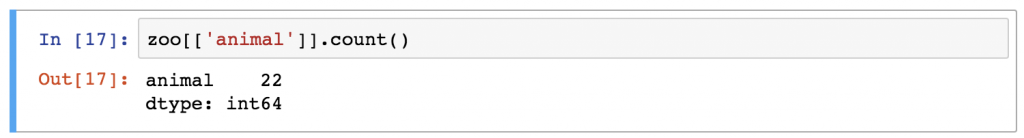Or in this particular case, the result could be even nicer if you use this syntax:

`zoo.animal.count()`

This also selects only one column, but it turns our pandas dataframe object into a pandas series object. (Which means that the output format is slightly different.)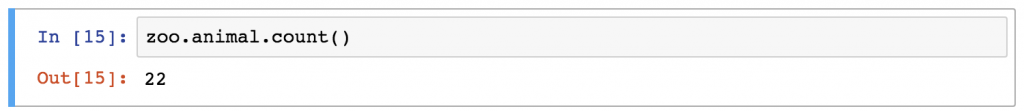## Pandas Data Aggregation #2: .sum()

Following the same logic, you can easily sum the values in the `water_need` column by typing:

`zoo.water_need.sum()`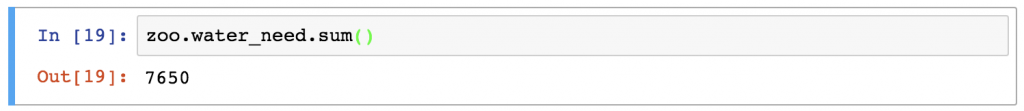Just out of curiosity, let’s run our sum function on all columns, as well:

`zoo.sum()`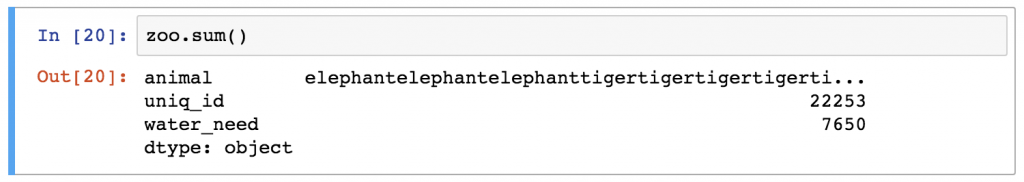Note: I love how `.sum()` turns the words of the `animal` column into one string of animal names. (By the way, it’s very much in line with the logic of Python.)

## Pandas Data Aggregation #3 and #4: .min() and .max()

What’s the smallest value in the `water_need` column? I bet you have figured it out already:

`zoo.water_need.min()`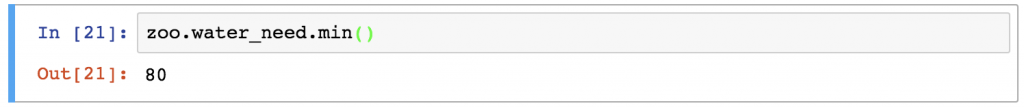And the max value is pretty similar:

`zoo.water_need.max()`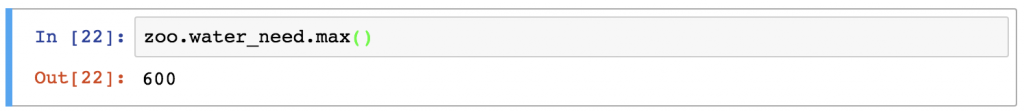## Pandas Data aggregation #5 and #6: .mean() and .median()

Eventually, let’s calculate statistical averages, like mean and median:

`zoo.water_need.mean()`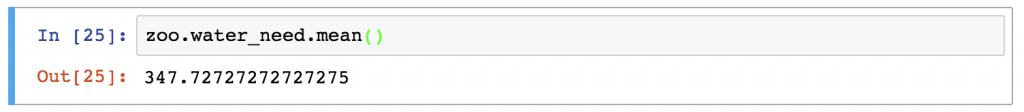`zoo.water_need.median()`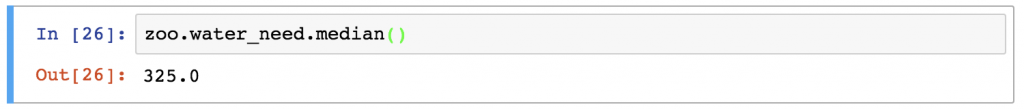Okay, this was easy. Much, much easier than the aggregation methods of SQL.
But let’s spice this up with a little bit of grouping!

## Grouping in pandas

As a Data Analyst or Scientist you will probably do segmentations all the time. For instance, it’s nice to know the mean `water_need` of all animals (we have just learned that it’s `347.72`). But very often it’s much more actionable to break this number down – let’s say – by animal types. With that, we can compare the species to each other – or we can find outliers.

Here’s a simplified visual that shows how pandas performs “segmentation” (grouping and aggregation) based on the column values!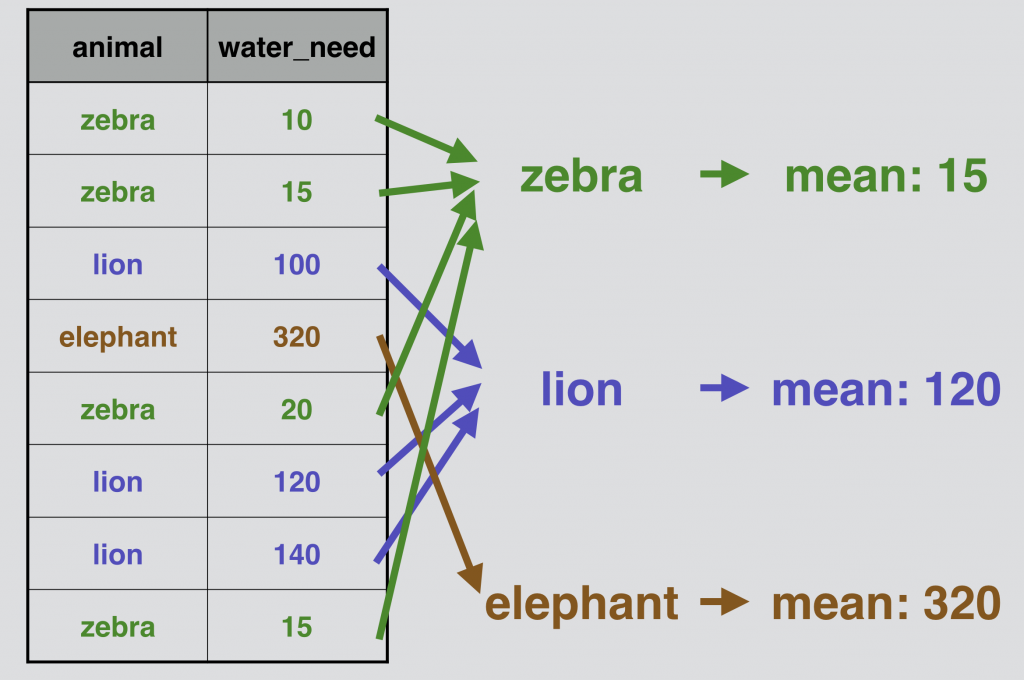## Pandas .groupby in action

Let’s do the above presented grouping and aggregation for real, on our `zoo` DataFrame!
We have to fit in a groupby keyword between our `zoo` variable and our `.mean()` function:

`zoo.groupby('animal').mean()`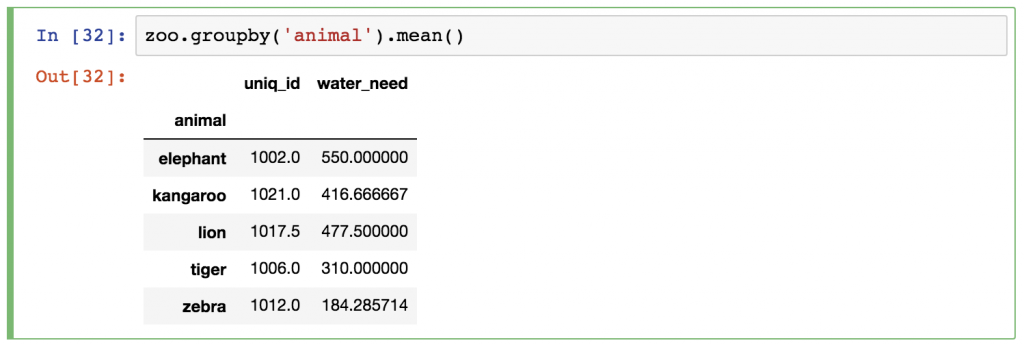Just as before, pandas automatically runs the `.mean()` calculation for all remaining columns (the `animal` column obviously disappeared, since that was the column we grouped by). You can either ignore the `uniq_id` column, or you can remove it afterwards by using one of these syntaxes:

`zoo.groupby('animal').mean()[['water_need']]` –» This returns a DataFrame object.

`zoo.groupby('animal').mean().water_need` –» This returns a Series object.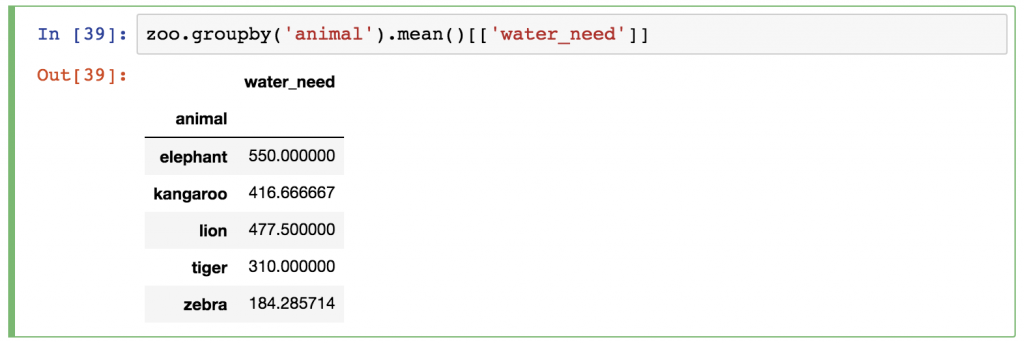Obviously, you can change the aggregation method from `.mean()` to anything we learned above!

Okay! Now you know everything, you have to know!
It’s time to…

## Test yourself #1

Let’s get back to our `article_read` dataset.

(Note: Remember, this dataset holds the data of a travel blog. If you don’t have the data yet, you can download it from here. Or you can go through the whole download, open, store process step by step by reading the previous episode of this pandas tutorial.)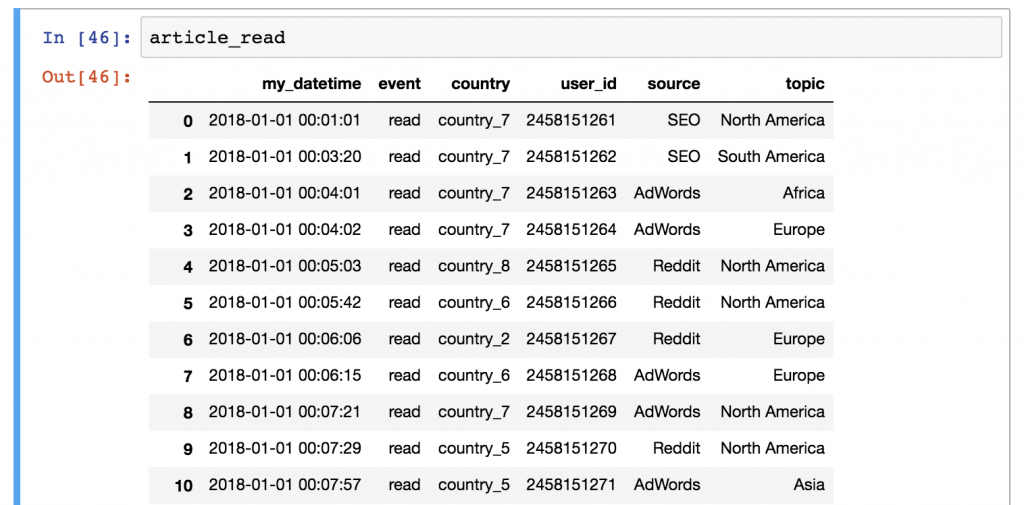If you have everything set, here’s my first assignment:

What’s the most frequent source in the `article_read` dataframe?
.
.
.
And the solution is: Reddit!
How did I get it? Use this code:

`article_read.groupby('source').count()`

Take the `article_read` dataset, create segments by the values of the `source` column (`groupby('source')`), and eventually count the values by sources (`.count()`).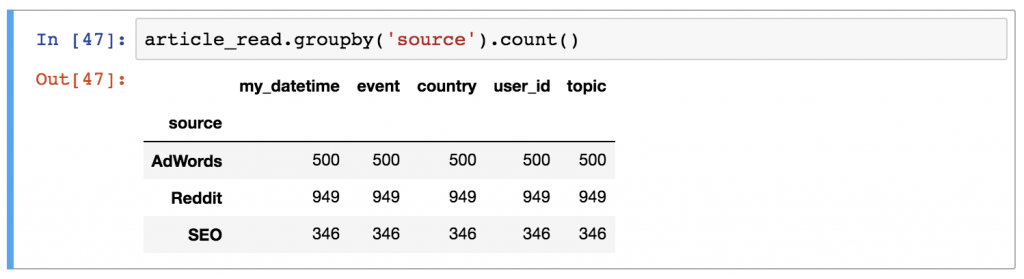You can – optionally – remove the unnecessary columns and keep the `user_id` column only:

`article_read.groupby('source').count()[['user_id']]`

## Test yourself #2

Here’s another, slightly more complex challenge:

For the users of `country_2`, what was the most frequent topic and source combination? Or in other words: which topic, from which source, brought the most views from country_2?
.
.
.
The result is: the combination of Reddit (source) and Asia (topic), with 139 reads!
And the Python code to get this results is:

`article_read[article_read.country == 'country_2'].groupby(['source', 'topic']).count()`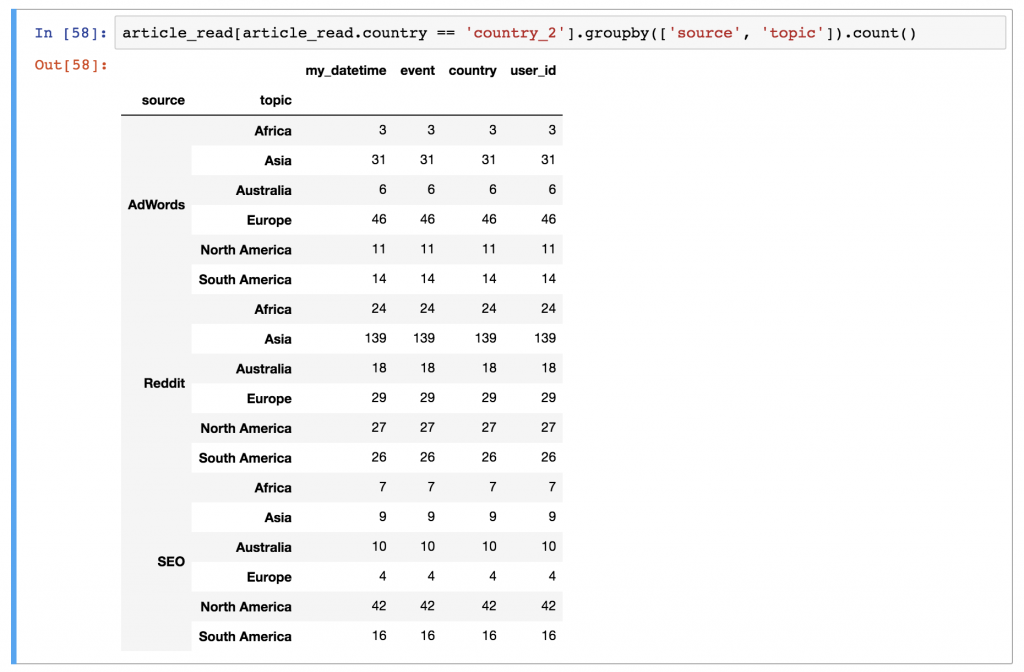Here’s a brief explanation:
First, we filtered for the users of `country_2` (`article_read[article_read.country == 'country_2']`). Then on this subset, we applied a `groupby` pandas method… Oh, did I mention that you can group by multiple columns? Now you know that! 😉 (Syntax-wise, watch out for one thing: you have to put the name of the columns into a list. That’s why the bracket frames go between the parentheses.) (That was the `groupby(['source', 'topic'])` part.)
And as per usual: the `count()` function is the last piece of the puzzle.

## Conclusion

This was the second episode of my pandas tutorial series. I hope now you see that aggregation and grouping is really easy and straightforward in pandas… and believe me, you will use them a lot!

Note: If you have used SQL before, I encourage you to take a break and compare the pandas and the SQL methods of aggregation. With that you will understand more about the key differences between the two languages!

In the next article, I’ll show you the four most commonly used “data wrangling” methods: `merge`, `sort`, `reset_index` and `fillna`. Stay with me: Pandas Tutorial, Episode 3!

Cheers,
Tomi Mester

1.•